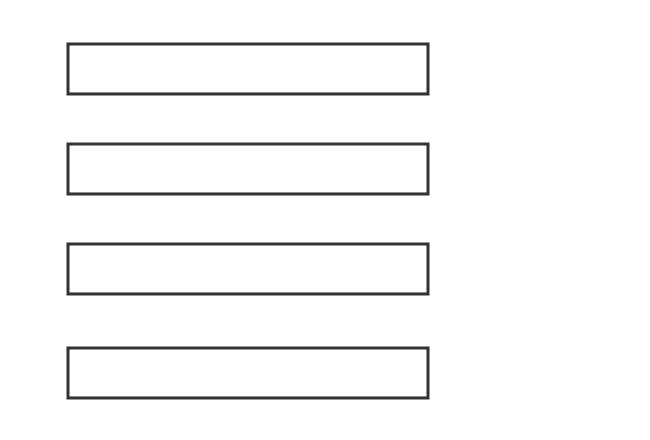# Equivalent fractions

The two or more fractions that have same value are called the equivalent fractions or equal fractions.

## Introduction

It seems, two or more fractions are dissimilar but if we calculate their values, they are equal surprisingly in some cases. So, the fractions are called as equivalent fractions and also simply called as equal fractions.

The fractions can be formed by the any number of equal divisions but they will be equal mainly due to their equality.

### Example

From a geometrical example, the concept of the equivalent fractions can be understood. Take four rectangles and split them as two, four, six and eight equal parts respectively.1. If we select a part as a fraction from first rectangle, then the fraction is equal to $\large \frac{1}{2}$
2. If we select two parts as a fraction from second rectangle, then the fraction is $\large \frac{2}{4}$
3. If we select three parts as a fraction from third rectangle, then the fraction is $\large \frac{3}{6}$
4. If we select four parts as a fraction from fourth rectangle, then the fraction is $\large \frac{4}{8}$

Thus, four proper fractions $\dfrac{1}{2}$, $\dfrac{2}{4}$, $\dfrac{3}{6}$ and $\dfrac{4}{8}$ are formed. In all four fractions, the numerators are different and denominators are different but their values are equal. Hence, the fractions are called as the equal fractions.

$\implies$ $\dfrac{1}{2}$ $\,=\,$ $\dfrac{2}{4}$ $\,=\,$ $\dfrac{3}{6}$ $\,=\,$ $\dfrac{4}{8}$ $\,=\,$ $0.5$

Similarly, the improper fractions can also form equivalent fractions. Therefore, the equal fractions can be either proper fractions or improper fractions but they both cannot be formed equivalent fractions.

Latest Math Topics
Jun 26, 2023
Jun 23, 2023

Latest Math Problems
Jul 01, 2023
Jun 25, 2023
###### Math Questions

The math problems with solutions to learn how to solve a problem.

Learn solutions

Practice now

###### Math Videos

The math videos tutorials with visual graphics to learn every concept.

Watch now

###### Subscribe us

Get the latest math updates from the Math Doubts by subscribing us.